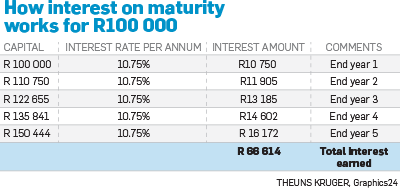Personal-Finance

# How to compare interest rates

2019-07-31 14:08You may have seen an advert for a five-year fixed deposit offering an interest rate of 13.3% on maturity. This seems like a really high interest rate, but what you need to understand is this is the interest you receive at the end of a five-year period. It is not the annual interest rate that is paid.

The simple interest rate, paid on maturity or expiry, includes interest you have earned on your interest by leaving it to be re-invested – allowing for a compounding effect. This means it is driven as much by the length of time you are invested as by the interest rate paid by the bank.

This rate on maturity is only valid if you leave your money fully invested, including all interest earned, for the full five years.

In this particular advert by African Bank the fine print reads that the interest rate is actually 10.75% per annum, which is equivalent to 13.33% per annum calculated on expiry after 60 months.

If the annual interest rate, compounded annually, is 10.75%, that means in one year a R100 000 investment would pay R10 750 in interest. But if you re-invest that interest the balance grows to R110 750 and the 10.75% interest is applied to this new, higher balance.

This results in more interest being earned in the second year of R11 905. If you reinvest that amount it grows the balance, and so forth.

If you re-invest R100 000 for five years, you would receive R66 600 in interest at the end of five years. By dividing that total rand interest earned by five years gives you R13 330 a year, or 13.3% per annum.While the annual compounded rate of 10.75% is still a good interest rate, and in fact currently the best rate offered over five years, it is not the same as the one grabbing the headline of the advert.

An annual compounded rate, also known as an annual effective or equivalent rate is the interest rate paid each year. Things get even more complicated when comparing interest rates, as they are stated as effective or nominal.

As interest is actually paid monthly, there is a compounding effect even within a year.

When you see “nominal”, that is the annual rate before the effect of monthly compounding, while “effective” is the interest rate when taking monthly compounding into effect.

So, for example, on a short period of one year you may see the following: “7.7% nominal and 7.97% effective”.

This means that the bank pays the interest monthly (7.7% divided by 12 months) so that each month the interest earns interest which means over a year your effective rate would be 7.97%.

For investment periods of less than a year, the bank will still show the nominal annual interest rate as if you were invested for the full year for comparative reasons, but your interest received would only be for the months you were invested – in other words, 7.7% divided by 12 months and multiplied by the number of months you are invested. For six months that would be 3.85% (7.7%/12 x 6 months).

The best way to compare rates is to compare the nominal interest rate, which is the “cleanest” comparison as it does not take the length of time invested into account.

In fact, if you calculate the nominal rate on the African Bank advert it works out to around 10.3%. You can then decide if that 10.3% is worth locking in for five years when you compare to other banks’ nominal rates.

Ideally you should ask the bank for a quote showing you the actual rand interest you will have received over the period of time you want to invest.

african bank
investment
interest rates

### Next on City PressDecember 6, 2019